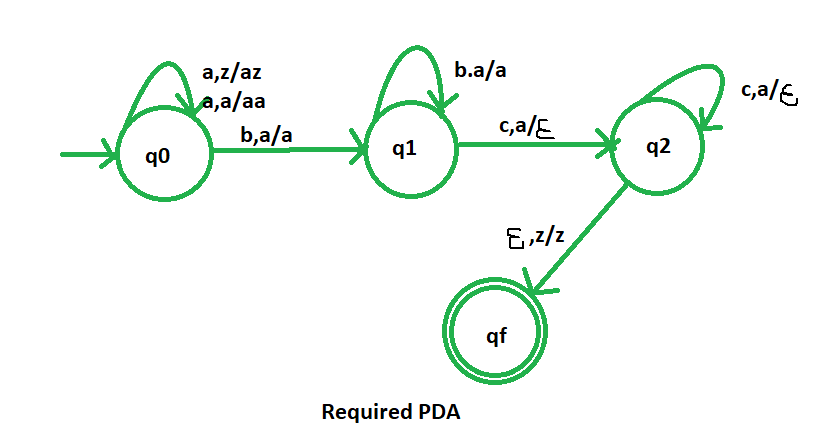# NPDA for accepting the language L = {an bm cn | m,n>=1}

Prerequisite – Pushdown automata, Pushdown automata acceptance by final state
Problem – – Design a non deterministic PDA for accepting the language L = {| m, n>=1}, i.e.,

 L = { abc, abbc, abbbc,  aabbcc, aaabccc, aaaabbbcccc, ...... }

In each of the string, the number of a’s is equal to number of c’s. And the number of b’s is independent of the number of a’s and c’s. This problem is quite similar to the NPDA for accepting the language L = {| m, n>=1}. The only difference is that here we useinstead of.

Explanation –
Here, we need to maintain the order of a’s, b’s and c’s.That is, all the a’s are are coming first and then all the b’s and then c’s are coming. Thus, we need a stack along with the state diagram. The count of a’s and c’s is maintained by the stack. The number of a’s is exactly equal to the number of c’s We will take 2 stack alphabets:= { a, z }


Where,= set of all the stack alphabet
z = stack start symbol

Approach used in the construction of PDA –
As we want to design a NPDA, thus every time ‘a’ comes before ‘b’. When ‘a’ comes then push it in stack and if again ‘a’ comes then also push it. And, when ‘c’ comes then pop one ‘a’ from the stack each time . And for ‘b’, we will do nothing in the stack only change the state in state diagram.
So, at the end if the stack becomes empty then we can say that the string is accepted by the PDA.

Stack transition functions –(q0, a, z)(q0, az)(q0, a, a)(q0, aa)(q0, b, a)(q1, a)(q1, b, a)(q1, a)(q1, c, a)(q2,)(q2, c, a)(q2,)(q2,, z)(qf, z )


Where, q0 = Initial state
qf = Final state= indicates pop operationSo, this is our required non deterministic PDA for accepting the language L = {| m, n>=1 }.My Personal Notes arrow_drop_upCheck out this Author's contributed articles.

If you like GeeksforGeeks and would like to contribute, you can also write an article using contribute.geeksforgeeks.org or mail your article to contribute@geeksforgeeks.org. See your article appearing on the GeeksforGeeks main page and help other Geeks.

Please Improve this article if you find anything incorrect by clicking on the "Improve Article" button below.